# Equilateral triangle

"Equilateral" redirects here. For other uses, see Equilateral (disambiguation).
Equilateral triangleType Regular polygon
Edges and vertices 3
Schläfli symbol {3}
Coxeter diagramSymmetry group D3
AreaInternal angle (degrees) 60°

In geometry, an equilateral triangle is a triangle in which all three sides are equal. In the familiar Euclidean geometry, equilateral triangles are also equiangular; that is, all three internal angles are also congruent to each other and are each 60°. They are regular polygons, and can therefore also be referred to as regular triangles.

## Principal properties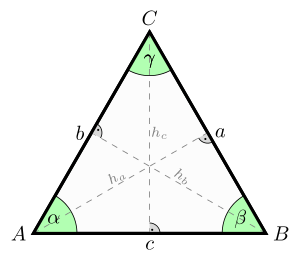An equilateral triangle. It has equal sides (a=b=c), equal angles (), and equal altitudes (ha=hb=hc).

Denoting the common length of the sides of the equilateral triangle as a, we can determine using the Pythagorean theorem that:

• The area is• The perimeter is• The radius of the circumscribed circle is• The radius of the inscribed circle isor• The geometric center of the triangle is the center of the circumscribed and inscribed circles
• And the altitude (height) from any side is.

Denoting the radius of the circumscribed circle as R, we can determine using trigonometry that:

• The area of the triangle isMany of these quantities have simple relationships to the altitude ("h") of each vertex from the opposite side:

• The area is• The height of the center from each side is• The radius of the circle circumscribing the three vertices is• The radius of the inscribed circle isIn an equilateral triangle, the altitudes, the angle bisectors, the perpendicular bisectors and the medians to each side coincide.

## Characterizations

A triangle ABC that has the sides a, b, c, semiperimeter s, area T, exradii ra, rb, rc (tangent to a, b, c respectively), and where R and r are the radii of the circumcircle and incircle respectively, is equilateral if and only if any one of the statements in the following nine categories is true. Thus these are properties that are unique to equilateral triangles.

### Sides

•••••### Semiperimeter

•••••### Angles

•••### Area

••••••### Equal cevians

Three kinds of cevians are equal for (and only for) equilateral triangles:

### Coincident triangle centers

Every triangle center of an equilateral triangle coincides with its centroid, which implies that the equilateral triangle is the only triangle with no Euler line connecting some of the centers. For some pairs of triangle centers, the fact that they coincide is enough to ensure that the triangle is equilateral. In particular:

### Six triangles formed by partitioning by the medians

For any triangle, the three medians partition the triangle into six smaller triangles.

• A triangle is equilateral if and only if any three of the smaller triangles have either the same perimeter or the same inradius.:Theorem 1
• A triangle is equilateral if and only if the circumcenters of any three of the smaller triangles have the same distance from the centroid.:Corollary 7

### Points in the plane

• A triangle is equilateral if and only if, for every point P in the plane, with distances p, q, and r to the triangle's sides and distances x, y, and z to its vertices,:p.178,#235.4## Notable theorems

Morley's trisector theorem states that, in any triangle, the three points of intersection of the adjacent angle trisectors form an equilateral triangle.

Napoleon's theorem states that, if equilateral triangles are constructed on the sides of any triangle, either all outward, or all inward, the centers of those equilateral triangles themselves form an equilateral triangle.

A version of the isoperimetric inequality for triangles states that the triangle of greatest area among all those with a given perimeter is equilateral.

Viviani's theorem states that, for any interior point P in an equilateral triangle with distances d, e, and f from the sides and altitude h,independent of the location of P.

Pompeiu's theorem states that, if P is an arbitrary point in an equilateral triangle ABC, then there exists a triangle with sides of lengths PA, PB, and PC. That is, PA, PB, and PC satisfy the triangle inequality that any two of them sum to at least as great as the third.

## Other properties

By Euler's inequality, the equilateral triangle has the smallest ratio R/r of the circumradius to the inradius of any triangle: specifically, R/r = 2.:p.198

The triangle of largest area of all those inscribed in a given circle is equilateral; and the triangle of smallest area of all those circumscribed around a given circle is equilateral.

The ratio of the area of the incircle to the area of an equilateral triangle,, is larger than that of any non-equilateral triangle.:Theorem 4.1

The ratio of the area to the square of the perimeter of an equilateral triangle,is larger than that for any other triangle.

If a segment splits an equilateral triangle into two regions with equal perimeters and with areas A1 and A2 , then:p.151,#J26If a triangle is placed in the complex plane with complex vertices z1, z2, and z3, then for either non-real cube rootof 1 the triangle is equilateral if and only if:Lemma 2Given a point P in the interior of an equilateral triangle, the ratio of the sum of its distances from the vertices to the sum of its distances from the sides is greater than or equal to 2, equality holding when P is the centroid. In no other triangle is there a point for which this ratio is as small as 2. This is the Erdős–Mordell inequality; a stronger variant of it is Barrow's inequality, which replaces the perpendicular distances to the sides with the distances from P to the points where the angle bisectors of ∠APB, ∠BPC, and ∠CPA cross the sides (A, B, and C being the vertices).

For any point P in the plane, with distances p, q, and t from the vertices A, B, and C respectively,For any point P on the inscribed circle of an equilateral triangle, with distances p, q, and t from the vertices,andFor any point P on the minor arc BC of the circumcircle, with distances p, q, and t from A, B, and C respectively,:170andmoreover, if point D on side BC divides PA into segments PD and DA with DA having length z and PD having length y, then:172which also equalsif tq; andwhich is the optic equation.

There are numerous triangle inequalities that hold with equality if and only if the triangle is equilateral.

An equilateral triangle is the most symmetrical triangle, having 3 lines of reflection and rotational symmetry of order 3 about its center. Its symmetry group is the dihedral group of order 6 D3.

Equilateral triangles are the only triangles whose Steiner inellipse is a circle (specifically, it is the incircle).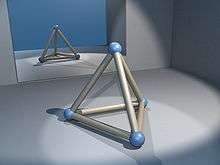A regular tetrahedron is made of four equilateral triangles.

Equilateral triangles are found in many other geometric constructs. The intersection of circles whose centers are a radius width apart is a pair of equilateral arches, each of which can be inscribed with an equilateral triangle. They form faces of regular and uniform polyhedra. Three of the five Platonic solids are composed of equilateral triangles. In particular, the regular tetrahedron has four equilateral triangles for faces and can be considered the three-dimensional analogue of the shape. The plane can be tiled using equilateral triangles giving the triangular tiling.

## Geometric construction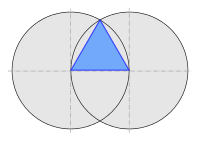Construction of equilateral triangle with compass and straightedge

An equilateral triangle is easily constructed using a compass and straightedge, as 3 is a Fermat prime. Draw a straight line, and place the point of the compass on one end of the line, and swing an arc from that point to the other point of the line segment. Repeat with the other side of the line. Finally, connect the point where the two arcs intersect with each end of the line segment

An alternative method is to draw a circle with radius r, place the point of the compass on the circle and draw another circle with the same radius. The two circles will intersect in two points. An equilateral triangle can be constructed by taking the two centers of the circles and either of the points of intersection.

In both methods a by-product is the formation of vesica piscis.

The proof that the resulting figure is an equilateral triangle is the first proposition in Book I of Euclid's Elements.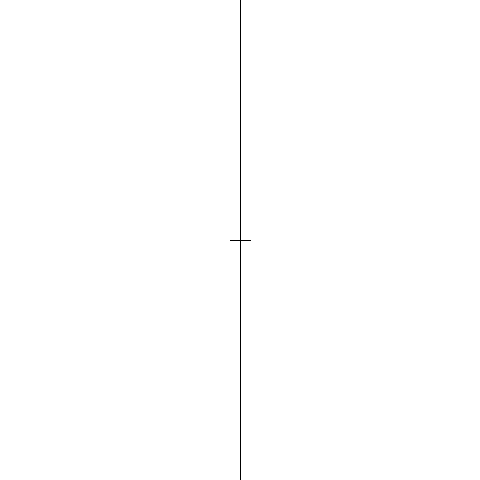## Derivation of area formula

The area formulain terms of side length a can be derived directly using the Pythagorean theorem or using trigonometry.

### Using the Pythagorean theorem

The area of a triangle is half of one side a times the height h from that side: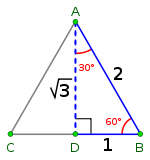An equilateral triangle with a side of 2 has a height of 3 as the sine of 60° is 3/2.

The legs of either right triangle formed by an altitude of the equilateral triangle are half of the base a, and the hypotenuse is the side a of the equilateral triangle. The height of an equilateral triangle can be found using the Pythagorean theoremso thatSubstituting h into the area formula (1/2)ah gives the area formula for the equilateral triangle:### Using trigonometry

Using trigonometry, the area of a triangle with any two sides a and b, and an angle C between them isEach angle of an equilateral triangle is 60°, soThe sine of 60° is. Thussince all sides of an equilateral triangle are equal.

## In culture and society

Equilateral triangles have frequently appeared in man made constructions:

## References

1. Andreescu, Titu; Andrica, Dorian (2006). Complex Numbers from A to...Z. Birkhäuser. pp. 70, 113–115.
2. Pohoata, Cosmin (2010). "A new proof of Euler's inradius - circumradius inequality" (PDF). Gazeta Matematica Seria B (3): 121–123.
3. Bencze, Mihály; Wu, Hui-Hua; Wu, Shan-He (2008). "An equivalent form of fundamental triangle inequality and its applications" (PDF). Research Group in Mathematical Inequalities and Applications. 11 (1).
4. Dospinescu, G.; Lascu, M.; Pohoata, C.; Letiva, M. (2008). "An elementary proof of Blundon's inequality" (PDF). Journal of inequalities in pure and applied mathematics. 9 (4).
5. Blundon, W. J. (1963). "On Certain Polynomials Associated with the Triangle". Mathematics Magazine. 36 (4): 247–248. doi:10.2307/2687913.
6. Alsina, Claudi; Nelsen, Roger B. (2009). When less is more. Visualizing basic inequalities. Mathematical Association of America. pp. 71, 155.
7. McLeman, Cam; Ismail, Andrei. "Weizenbock's inequality". PlanetMath.
8. Owen, Byer; Felix, Lazebnik; Deirdre, Smeltzer (2010). Methods for Euclidean Geometry. Mathematical Association of America. pp. 36, 39.
9. Yiu, Paul (1998). "Notes on Euclidean Geometry" (PDF).
10. Cerin, Zvonko (2004). "The vertex-midpoint-centroid triangles" (PDF). Forum Geometricorum. 4: 97–109.
11. Chakerian, G. D. "A Distorted View of Geometry." Ch. 7 in Mathematical Plums (R. Honsberger, editor). Washington, DC: Mathematical Association of America, 1979: 147.
12. Posamentier, Alfred S.; Salkind, Charles T. (1996). Challenging Problems in Geometry. Dover Publ.
13. Svrtan, Dragutin; Veljan, Darko (2012). "Non-Euclidean versions of some classical triangle inequalities" (PDF). Forum Geometricorum. 12: 197–209.
14. Dörrie, Heinrich (1965). 100 Great Problems of Elementary Mathematics. Dover Publ. pp. 379–380.
15. Minda, D.; Phelps, S. (2008). "Triangles, ellipses, and cubic polynomials". American Mathematical Monthly. 115 (October): 679–689.
16. Dao, Thanh Oai (2015). "Equilateral triangles and Kiepert perspectors in complex numbers" (PDF). Forum Geometricorum. 15: 105–114.
17. Lee, Hojoo (2001). "Another proof of the Erdős–Mordell Theorem" (PDF). Forum Geometricorum. 1: 7–8.
18. De, Prithwijit (2008). "Curious properties of the circumcircle and incircle of an equilateral triangle". Mathematical Spectrum. 41 (1): 32–35.

Fundamental convex regular and uniform polytopes in dimensions 2–10
Family An Bn I2(p) / Dn E6 / E7 / E8 / E9 / E10 / F4 / G2 Hn
Regular polygon Triangle Square p-gon Hexagon Pentagon
Uniform polyhedron Tetrahedron OctahedronCube Demicube DodecahedronIcosahedron
Uniform 4-polytope 5-cell 16-cellTesseract Demitesseract 24-cell 120-cell600-cell
Uniform 5-polytope 5-simplex 5-orthoplex5-cube 5-demicube
Uniform 6-polytope 6-simplex 6-orthoplex6-cube 6-demicube 122221
Uniform 7-polytope 7-simplex 7-orthoplex7-cube 7-demicube 132231321
Uniform 8-polytope 8-simplex 8-orthoplex8-cube 8-demicube 142241421
Uniform 9-polytope 9-simplex 9-orthoplex9-cube 9-demicube
Uniform 10-polytope 10-simplex 10-orthoplex10-cube 10-demicube
Uniform n-polytope n-simplex n-orthoplexn-cube n-demicube 1k22k1k21 n-pentagonal polytope
Topics: Polytope familiesRegular polytopeList of regular polytopes and compounds